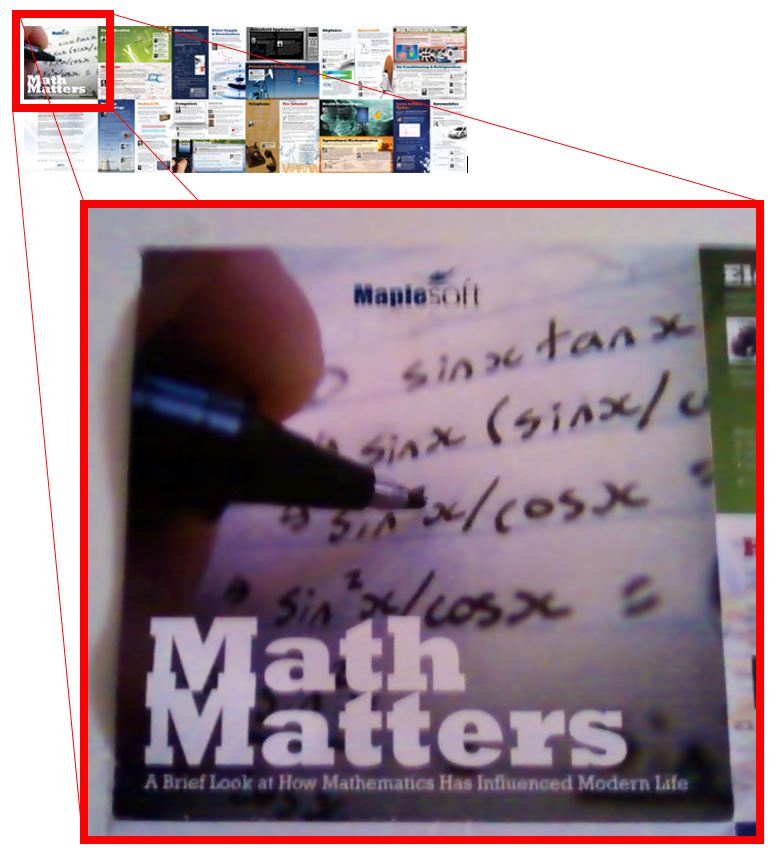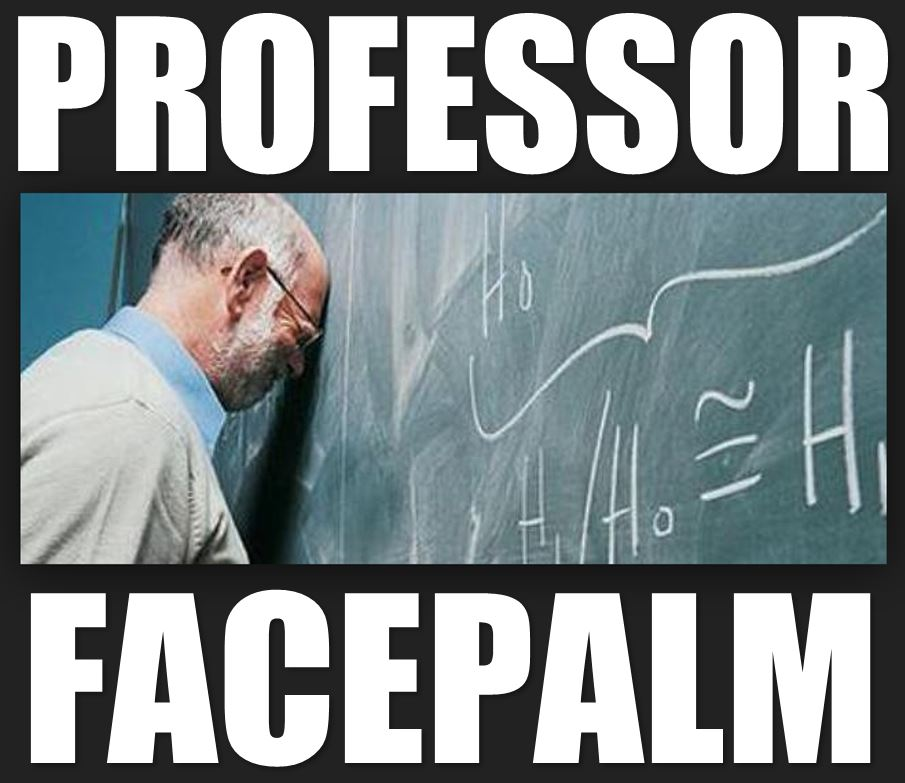# Every story has a beginning, middle, and end… just not necessarily in that order

One of my gripes with posters that are supposed to be “mathy” is not that they fall into the same common cliche of a slightly frazzled-looking nerd chugging his or her way through a sea of equations at a blackboard… just because it’s a cliche doesn’t mean it’s not accurate.  Nor is it that aforementioned sea of mathematics is typically a sea of nonsense characters cobbled together from completely unrelated branches of mathematics in no discernible logical order… the point is to look impressive and daunting, not to prove the Goldbach conjecture.

No, my main gripe is the one illustrated in these several examples I found below:The problem?  This is not how you math.

No one writes out a paragraph of equations from beginning to end and then goes back and fills in all the subscripts or superscripts or numerators.  No one, for example, computes the derivative of$sin^3 x^5$ by first writing$displaystyle frac{d}{dx} sin x = 3 cdot sin x cdot cos x cdot 5 x$

on their first pass, and then goes back to fill that in with$displaystyle frac{d}{dx} sin^3 x^5 = 3 cdot sin^2 x^5 cdot cos x^5 cdot 5 x^4$.

Hence, one would expect that a mathematical company, say MapleSoft, attempting to sell a mathematical product, say Maple, to a mathematically-proficient community, say engineering schools, would not fall into such traps.  Like, say, this one:In case you can’t see it,All I can say…